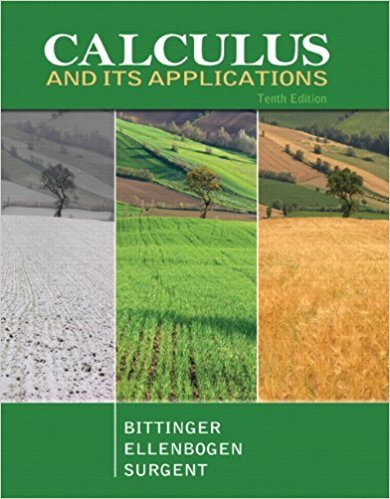×
×

# Solutions for Chapter 5.1: An Economics Application: Consumer Surplus and Producer Surplus## Full solutions for Calculus and Its Applications | 10th Edition

ISBN: 9780321694331Solutions for Chapter 5.1: An Economics Application: Consumer Surplus and Producer Surplus

Solutions for Chapter 5.1
4 5 0 406 Reviews
31
2
##### ISBN: 9780321694331

This textbook survival guide was created for the textbook: Calculus and Its Applications, edition: 10. Chapter 5.1: An Economics Application: Consumer Surplus and Producer Surplus includes 21 full step-by-step solutions. Calculus and Its Applications was written by and is associated to the ISBN: 9780321694331. Since 21 problems in chapter 5.1: An Economics Application: Consumer Surplus and Producer Surplus have been answered, more than 25036 students have viewed full step-by-step solutions from this chapter. This expansive textbook survival guide covers the following chapters and their solutions.

Key Calculus Terms and definitions covered in this textbook
• Absolute value of a complex number

The absolute value of the complex number z = a + b is given by ?a2+b2; also, the length of the segment from the origin to z in the complex plane.

• Annuity

A sequence of equal periodic payments.

• Constant term

See Polynomial function

• Demand curve

p = g(x), where x represents demand and p represents price

• Half-plane

The graph of the linear inequality y ? ax + b, y > ax + b y ? ax + b, or y < ax + b.

• Inverse composition rule

The composition of a one-toone function with its inverse results in the identity function.

• Inverse sine function

The function y = sin-1 x

• Modulus

See Absolute value of a complex number.

• Open interval

An interval that does not include its endpoints.

• Pythagorean

Theorem In a right triangle with sides a and b and hypotenuse c, c2 = a2 + b2

• Reflection through the origin

x, y and (-x,-y) are reflections of each other through the origin.

• Row echelon form

A matrix in which rows consisting of all 0’s occur only at the bottom of the matrix, the first nonzero entry in any row with nonzero entries is 1, and the leading 1’s move to the right as we move down the rows.

• Sample survey

A process for gathering data from a subset of a population, usually through direct questioning.

• Sphere

A set of points in Cartesian space equally distant from a fixed point called the center.

• Standard form of a polar equation of a conic

r = ke 1 e cos ? or r = ke 1 e sin ? ,

• Standard position (angle)

An angle positioned on a rectangular coordinate system with its vertex at the origin and its initial side on the positive x-axis

• Subtraction

a - b = a + (-b)

• Sum of a finite arithmetic series

Sn = na a1 + a2 2 b = n 2 32a1 + 1n - 12d4,

• Weights

See Weighted mean.

• Work

The product of a force applied to an object over a given distance W = ƒFƒ ƒAB!ƒ.

×